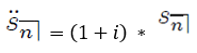Search
•Kalpesh Agrawal

# Annuity-Due and its formula

Let's understand Annuity-Due.

• An Annuity-Due is an annuity for which the payments are made at the beginning of the payment periods.

• The first payment is made at time 0, and the last payment is made at time n-1.

• The present value of the annuity-due at time 0 by:• The future value of the annuity at time n by:• The formula for the Present and future value of an annuity due is as follows:• Each payment in an annuity due is paid one period ahead of the corresponding payment of an annuity-immediate. We can write the formula for Annuity due in Annuity Immediate terms as follows:Written by: Kalpesh Agrawal (Jr. Actuarial Officer)